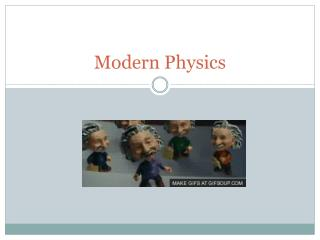DownloadDownload PresentationModern Physics

# Modern Physics

Download Presentation## Modern Physics

- - - - - - - - - - - - - - - - - - - - - - - - - - - E N D - - - - - - - - - - - - - - - - - - - - - - - - - - -
##### Presentation Transcript

1. Modern Physics

2. Fukushima News 3/18/13 – BBC Link

3. Atom PHET Atom Simulation - Link

4. Atom Nucleons – subatomic particles in the atom’s nucleus (protons and neutrons). Ion – An atom with a net electric charge which is due to the loss or gain of electrons. Strong nuclear force – Nucleons attractive binding force.

5. 38.2Light Quanta Einstein visualized particles of light as concentrated bundles of electromagnetic energy. Max Planck had proposed that atoms do not emit and absorb light continuously, but do so in little chunks. Each chunk was considered a quantum, or a fundamental unit.

6. 38.2Light Quanta Planck believed that light existed as continuous waves, but that emission and absorption occurred in quantum chunks. Einstein went further and proposed that light itself is composed of quanta. One quantum of light energy is now called a photon.

7. Photoelectric Effect - Link The energy of a photon is directly proportional to the photon’s frequency. Planck’ constant (h) – A fundamental constant of quantum theory that determines the scale of the micro world. E = h f E = photon energy h = Planck’s constant f = frequency

8. 38.2Light Quanta • The energy of a photon of light is proportional to its vibrational frequency. • When the energy E of a photon is divided by its frequency f, the quantity that results is known as Planck’s constant, h. • This quantity is always the same, no matter what the frequency. • The energy of every photon is therefore E = hf. • This equation gives the smallest amount of energy that can be converted to light of frequency f.

9. 38.3The Photoelectric Effect Einstein found support for his quantum theory of light in the photoelectric effect. The photoelectric effect is the ejection of electrons from certain metals when light falls upon them. These metals are said to be photosensitive. PHET SIMULATION LINK

10. 38.8Quantum Physics Physicists became convinced that the Newtonian laws that work so well for large objects do not apply to the microworld of the atom. The study of the motion of particles in the microworld of atoms and nuclei is called quantum mechanics. The branch of physics that is the general study of the microworld of photons, atoms, and nuclei is simply called quantum physics.